Home
Web--Site--Page--List

## Gravitational Constants and Pressures

 < Prev Pg Double Force Page Contents Site Page List < Site Contents Bottom Next Pg > Create Energy

Abstract:    This is a public domain article.

•  The Gravity Maximum, of our Solar System:....All planetary bodies larger than Earth exhibit the maximum gravity of:
1.141 g, ....= 11.9 meters / sec^2, ....= 1.141 gram force / gram.
• The Gravitational Shielding Limit, Densare Max:....The gravitational shielding limit, Densare Max, is the minimum amount of matter per unit area that will absorb all gravitational radiation. The gravitational shielding, Densare, for all planets is obtained by dividing the planets apparent mass by the cross-sectional area.
• The Radiation Pressure Limit, A Solar System constant:....The maximum potential radiation pressure for a planet is obtained by multiplying the surface gravity by the shielding value, Densare Max, which will stop all gravitational radiation
• Our Gravitational Constant, "G":.... is derived using only the radiation and shielding concept and the features of our Solar system, it will differ in other solar systems and will be proportional to their maximum radiation pressure.
• Q. Majorana's Absorption Constant for Gravity:.... is reviewed and replicated, using Solar system data. The resulting value of gravitational absorption found by this study is approximately " 23 " times larger than that found by Dr. Majorana's experiments of circa 1920 AD.
• The Sun's true effective Mass:....can not exceed 4 % of NASA's Standard Model value. The Sun's large magnetic field shields gravitational radiation making the Sun's mass and Densare appear greater than actual. It is possible that the material of the visible Sun is completely shielded by the central magnetic field and the Sun does not contribute to the solar central gravitational force. The measurable  gravitational shielding by the large magnetic fields demonstrate the causal unification of gravitational, inertial and electromagnetic forces via a radiation and shielding force system.
• There are no gas planets:....The large planets consist of a gravel mix with a density comparable to the density of the smaller planets. Their "apparent" small weight and inertia is attributed to the shielding of the bulk of the deep interior matter from prime radiation. Prime radiation and shielding in this model are the cause of gravitation: i.e. effective mass, weight and inertia.

***                                                                  GvR 1

 Page Contents:     Gravitational Pressure and Constants

The content in this article authored by me is granted to be in the public domain,  distribute and translate freely.  Cheers.   Signed:  Stanley V Byers  10/23/2014

 < Prev Pg Double Force Page Contents Site Page List < Site Contents Bottom GvR 2

### The Gravity Maximum

The Gravity Maximum, a physical constant of our Solar System. All planets, larger than Earth exhibit the maximum gravity of 1.141 g,  = 11.9 meters / sec^2,  = 1.141 gram force / gram.

Gravity and Radiation Pressure Limits occur at Total Shielding. From Fig. 1 above, it is seen that radiation shielding and gravity are essentially proportional to the diameter of small planets and moons. When celestial objects become large enough,... all radiation is blocked and the pressure and gravity become limited at the common maximum value. All solar system objects larger than Earth exhibit total shielding and therefore display the common maximum gravity of 1.141g,  or  11.9 m/sec^2.         Continued

 < Prev Pg Double Force Page Contents Site Page List < Site Contents Bottom GvR 3

The common gravity limit of 1.141 g,...indicates that hypothetical Black Holes and Neutron Stars can not exist in a radiation and shielding system. Black Holes and Neutron Stars are hypothetical features of the Mass Attraction and General Relativity attraction theories. The paper "Force Doubling Paradox of Gravitational Attraction" provides evidence showing that attraction models of gravitation are not realistic.

Saturn, the largest solar system object without an effective magnetic field shield, is used as the gold standard for establishing and calibrating the constants:

• Gravity Maximum
• Densare Shielding Maximum

The surface gravity for Saturn is determined by the orbital force of it's moons. The Orbital force of each moon indicates that Saturn's gravity is 1.141 g, = 1.141 gram force per gram. NASA's published value for Saturn's gravity is 1.061 gram force per gram.        ***

### The Gravitational Shielding Limit, Densare Max.

The gravitational shielding Densare for all planets is produced by dividing the planets apparent mass by the cross-sectional area.
For the large black shadow planets the apparent weight, mass and inertia are no longer proportional to volume but are exactly proportional to the cross sectional area (Ax). The numerical quantity of atoms is the only attribute that remains proportional to volume.

From Fig.1 above the common shielding Densare limit is evident for all planets larger than earth. The maximum shielding value is 5.3366×10^9 grams per square centimeter.

In the original DENSARE Graph    Fig. 1 , Jupiter is shown to have an anomalous shielding value approximately 3 times greater than the maximum value for black shadow planets. However, the actual shielding of Jupiter is the same as the other black shadow planets. The anomalous value is due to the cross-sectional area of the large unseen magnetic field surrounding Jupiter, which also shields gravitational radiation and thereby provides additional apparent mass to Jupiter's position.  ***

 < Prev Pg Double Force Page Contents Site Page List < Site Contents Bottom GvR 4

### The Radiation Pressure Limit,   A Solar System constant.

The maximum potential radiation pressure for a planet is obtained by multiplying the surface gravity by the shielding value, Densare Max, which will stop all gravitational radiation. This same method is used to find the reduced pressure values for the smaller planets.

Saturn's radiation pressure equals the Radiation Pressure Limit, a constant of our solar system.       Given:   1g = 1 gram force / gram

Pr_sa = ( g_sa ) gf/gm x ( Dn_mx ) gm/cm^2

Pr_sa = 1.141 g x 5.3366 x 10^9 gm/cm^2

Pr_sa = Pr_mx = 6.09 x 10^6 kgf / cm^2

Pr_sa = 86.6 million PSI,  = Pr_max,   =  600 GPa  Approx.

The potential radiation pressure for each of the black shadow planets is equal to this radiation pressure limit. This radiation pressure limit, Pr_max, a constant derived in our solar system.   ***

### A Gravitational Constant, " G ",   derived via Radiation Pressure

The Gravitational Constant, "G" is derived using only the radiation and shielding concept and the known physical features of the Solar system as provided by NASA data. This is not an attempt to improve or contest the accuracy of the published conventional gravitational constants.
The goal is to demonstrate and validate a radiation and shielding system of gravitation by correctly reproducing the standard gravitational constant using the radiation pressure concept only, without mass attraction and GR attraction.
The Gravitational constant derived here, with the radiation pressure system of gravity, matches NASA's derived value within 2 parts in 10,000 parts.

It will be derived by comparing the gravitational radiation flow and pressure towards the Earth,...to the equal opposing orbital force of the Moon. It is clear that the acceleration of the Moon toward the Earth is an effect directly caused by radiation flow and potential pressure towards the Earth. The Moon's opposing orbital centrifugal reaction force is used to gauge the effective value of the Earth's potential pressure (gravity) at the Moon.

Since the gravitational constant is shown here to be directly proportional to the maximum radiation pressure it has to be considered a variable, that will depend on the gravitational pressure within a particular solar system. This indicates that our gravitational constant cannot be used for calculations with in other solar systems.

The Moon's orbital force ( Fc = M * v^2 / r ) is found by using the Moon's mass (M_mn), orbital velocity (v_mn) and the orbit's radius to the barycenter (yb_mn) as givens from NASA. This orbital inertial reaction force is equal to and balances the radiation force, ( g ) toward the Earth.

 < Prev Pg Double Force Page Contents Site Page List < Site Contents Bottom GvR 5

This math derivation, using only NASA's data and Mathcad 15, produces:

• The Orbital force of the Moon,   Fc_mn , in Kgf
• The Earth's potential radiation press.,  Pr_e , in gf / cm^2 .
• The Earth's gravitational force per gram, ( g ) in gf / gm,  and
• The gravitational constant,  ( G )

The Earth's gravity ( g ), is derived without using Newton's equation, and without using the gravitational constant ( G ).  Ref.:  Addendum C:  Math Assumptions

 Deriving a Gravitational Constant     Givens:   NASA data for Earth's Moon One g = one gram force / gram mass 1 g = 1gf / gm Mass of Moon,  M_mn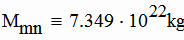Projected Area of Moon, Effective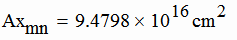Orbital Velocity, v_mn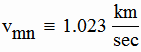Radius to barycenter,   yb_mn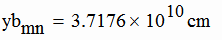Distance between Earth / Moon centers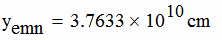Moon's Shadow density, Densare, Dn_mn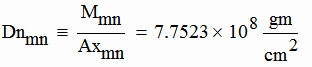One "g" per NASA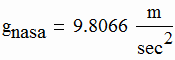Shadow density value ( Dn_max ) which stops all Gravitational Radiation,...a physical constant in our solar system.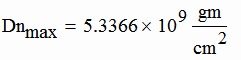Earth's:   Mass               Radius M_e = 5.9736 x 10^24 kg r_e = 6.3761 x 10^6 meters

### Deriving the  Gravitational Constant via Radiation Pressure

Moon's Orbital Force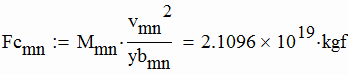Earth's "Effective"  Rad. Press. towards Earth at Moon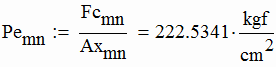Moon's Shielding ratio  %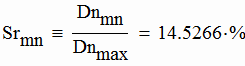Earth's "Potential" Pressure at Moon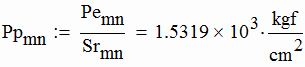1 ) Potential Radiation Pressure at Earth,       75.9 meg psi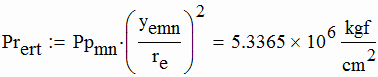The next step uses the max shielding, Dn_max to shield 100% of Earth's radiation pressure.
2 ) Derived (g) in gram force per gram mass (gf / gm ).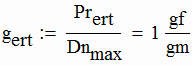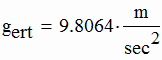3 ) G via g.ert
Derivation via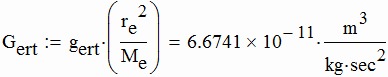< Prev Pg Double Force Page Contents Site Page List < Site Contents Bottom GvR 7

#### Comparing this Gravitational Constant, "G"with other values of "G" .

3 ) Gravitational constant "G"

Units of gravity / shielding  > >

Units of gravity / shielding  > >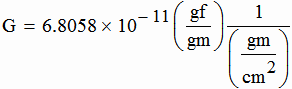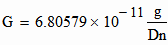1)   G from NASA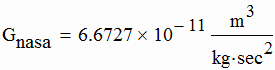2)   G from Britannica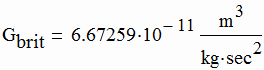3)   G from Wikipedia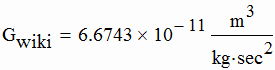4)   G from Mathcad 15 Data files.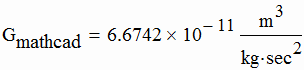Resulting Accuracy:    Derived "G" is: 99.97 % of the avg. of the set of 4 values above, 99.98 % of the value published by NASA. The max. difference in the above data set of 4 is approx: 2.5 parts in 10 thousand The max. difference:   NASA's "G" vs the derived "G" is: 2 parts in 10 thousand.

Web Link to NASA Planets Data.      http://solarsystem.nasa.gov/planets/profile.cfm?Object=Uranus         ***

 < Prev Pg Double Force Page Contents Site Page List < Site Contents Bottom GvR 8

### Q. Majorana's Gravitational Absorption Constant

Q. Majorana's Absorption Constant for Gravity is reviewed and replicated, using Solar System data, and a radiation and shielding model of gravity. The resulting value of the gravitational absorption ratio found by this study is approximately 23 times larger than the value found by Dr. Majorana's experiments of circa 1920 AD.

Q. Majorana's excellent vision and shielding work of circa 1920 AD, was first and he was first to publish and was correct in demonstrating that gravitational shielding exists. Except for one small error it appears that he would have found the same larger shielding rate that is now evidenced by this work via a radiation and shielding system of gravity.

Majorana apparently assumed that gravity could be due to:
1) external radiation pressure, " or " 2) attraction.
As a result, he provided shielding both above and below the test mass on all tests.
Current absorption studies using a radiation and shielding system indicate that if Majorana had placed a shield only above the test mass, he could have found the absorption ratio to be 23 times larger than his published data.

In this radiation and shielding model, the coherency and the shielding of the prime radiation are the main causes of gravity. When the coherent radiation encounters and penetrates the planet it is not absorbed, it is reflected from the internal atoms and nucleons and scattered. The gravitational force decreases with depth not because of absorption, but because the effective coherent radiation is scattered via reflection into ineffective in-coherent radiation. The reflected radiation is obviously spatially incoherent, since it is diverging from a multitude of point sources.
Majorana's absorption constant can also be called Majorana's shielding rate.

Gravitation's reduction with depth follows an exponential curve because the loss is proportional to the reduced radiation value at depth. Intuition erroneously suggests that the absorption constant would remain constant for all planet sizes. However, planet data reveals that the absorption constant is not constant, it increases by approximately 20% for Earth and Venus in relation to the moon and small planets, Fig. 2. All of the large black shadow planets have the same maximum absorption rate which is 47 % greater than the rate for the small moons and planets.  A portion of the reflected radiation apparently interferes with the incoming radiation force and thus increases the static shielding rate. This reflection feature accounts for the linear increase of the absorption ratio with planet size.

 < Prev Pg Double Force Page Contents Site Page List < Site Contents Bottom GvR 9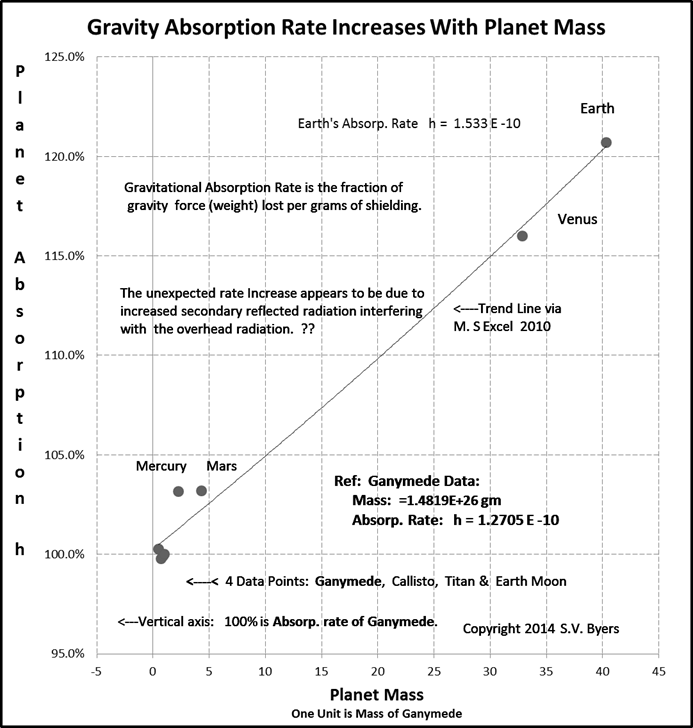Gravitational Absorption vs Planet Mass
Figure 2

In this model the incoherent reflected prime radiation becomes coherent again as it completes its cycle by returning to merge with the coherent radiation of outer space. A closed cycle removes the concern that the prime radiation may be consumed through absorption.        Continued

 < Prev Pg Double Force Page Contents Site Page List < Site Contents Bottom GvR 10

A physical and mathematical analog of gravitational radiation absorption (shielding) exists in the study of sunlight being absorbed as it penetrates into lake water. Addendum B: Shielding by Lake Water   If one slowly submerges a light meter into a clear lake, it will be found that approximately 1/2 of the available light intensity will be absorbed by a depth of 15 feet. The exponential decay equation for describing the light intensity degradation with depth is directly applicable to gravitational radiation force degradation with depth. Wikipedia provides a review of the natural exponential decay process and the required equations at :     http://en.wikipedia.org/wiki/Exponential_decay

 Gravitational Absorption via Exponential Decay           Givens:  Derived from NASA data Addendum C provides a list of the simplifying assumptions made for the systems unknown physical properties used in the Mathcad work presented here. Pressure Radiation MaximumSaturn's gravity times it's Shielding Densare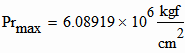Pressure Radiation Earth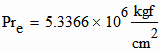Remaining Unshielded Pressure Earth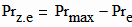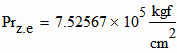Absorption rate   per centimeter,   Lamda, (Lm) Lm.e = h x rho.e Absorption rate per gram h.e = Lm.e / rho.e Density of Earth materialper Radiation Pressure Model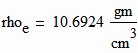Diameter of Earth,  D_e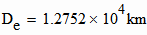Mass of Earth, M.e.   Nasa Data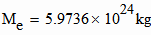Shielding:   Units of Densare,  (Dn) 1 x Dn = 1 gm/cm^2

 < Prev Pg Double Force Page Contents Site Page List < Site Contents Bottom GvR 11

#### Gravitational absorption via Exponential Decay Ref. Givens above, via Math Cad 15.

Earth's Rad. Press. with Depth,   Pr_i.e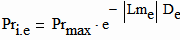For the earth and small planets the surface pressure and the exit pressure are readily known. With two known points on the exponential decay profile, the curve and the absorption rate are readily obtained.
Dividing through by Pr_max and taking the natural log of each side provides the equation for obtaining the absorption ratio per depth, 1/(cm)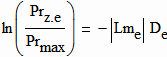Earth's Average Absorp. ratio per depth.  Lm.e 1/cm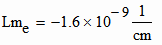Earth's Absorp. ratio,  h.e  in gram loss per gram / cm^2 will be derived in the next section.

### Earth's avg. effective density and Earth's absorption ratio per gram via the radiation and shielding system.

The exponential equation work given above only produces an absorption rate per depth. Majorana's name for the per depth rate is lambda, the symbol used is Lm.
In order to obtain the absorption rate per gram from the Lambda rate, the effective density of the material must be found. Majorana knew the density of his shielding  material, but the Earth's average effective density for a radiation shielding system is unknown and may be found with the following mathematics.

 < Prev Pg Double Force Page Contents Site Page List < Site Contents Bottom GvR 12

 Absorption Rate per Gram:   h.e           Ref:  Givens provided above The "Layer Cake" formula for Earth Mass.    Ref:  Wikipedia disk integration info:     https://en.wikipedia.org/wiki/Disk_integration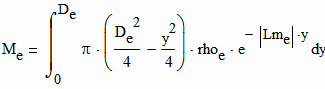Integrating these above terms over the diameter  of the Earth gives the volume.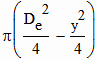These terms provide the proper "density" for each layer of integration. Assuming a homogenous mix of atoms.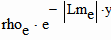The only unknown in the M.e equation is effective density, rho.e.    Increasing rho.e to 10.69 gm/cm^3 provides the Earth's known mass of:  Earth,  NASA given:  M.e = 5.9736 x 10 ^24 kg M.e = 5.9736 x 10 ^24 kg      therefore   rho.e = 10.69 gm/cm^3 Inserting rho.e into the Lamda equation provides Earth's absorption ratio per  gram ,  h.e h.e = Lm.e / rho.e h.e = 1.53 x 10^-10    2014 AD Q Majorana's Absorption Rate,  h.qm        1920 AD  vs   2014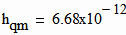1920 AD h.e / h.qm = 23

Using Dr. Q. Majorana's equations, a radiation and shielding system and NASA's data,... Majorana's absorption rate has been updated to:   h = 1.53 x 10E(-10) .     ***

 < Prev Pg Double Force Page Contents Site Page List < Site Contents Bottom GvR 13
////////////////////////

### Gravimeter Ring Shielding Results

Within a radiation and shadowing system of gravity, a free body in free space is bathed by an equal amount of radiation force from all sides, which gives an isotropic force balance condition. As a free body ventures closer to a planet it would be shielded from a portion of the radiation from the direction of the planet. Since the free space balanced radiation force is now unbalanced, an acceleration toward the planet will result. Once in a stable orbit the body's outward centrifugal inertial force balances the radiation pressure towards the planet's position.
In studies of the past it was assumed that gravitational force arose as a physical action (attraction) that occurred only in the space between the two bodies of mass. With that assumption it seemed reasonable that a shield between the two bodies would decrease the gravitational force.
However, within the geometry and physical mechanics of a radiation and shadowing system it is obvious that an additional shield beneath a test mass will increase the measured weight of the test mass by adding to the density of the planets gravity shadow. This increase of force is clearly demonstrated by the measurements taken for two gravimeter calibration test programs of the 1990's.

The following quote is a short description of the calibration method used by the 1994 and 1997 calibration tests preformed by G. Casula, et al.

Quote   In our station a new calibration method is used:  it consists in moving a mass of simple geometry (  circular ring with square cross section  ), along the vertical axis of the instrument, in order to perturb ( Shield ) the gravity field in a known way, ( Achilli et al., 1995; Varga et al,. 1995 )    Unquote

### False Data using "Mass Attraction"

It is possible to generate the theoretical affect curve in the following chart using Newton's equation and the mass attraction concept. However; The paper "Force Doubling Paradox of Gravitational Attraction" adequately demonstrates that mass attraction is not a realistic concept.

 < Prev Pg Double Force Page Contents Site Page List < Site Contents Bottom GvR 14

Figure 3, below, is a chart of the calibration measurements using the circular ring method.  (Chart's notes are by current author.)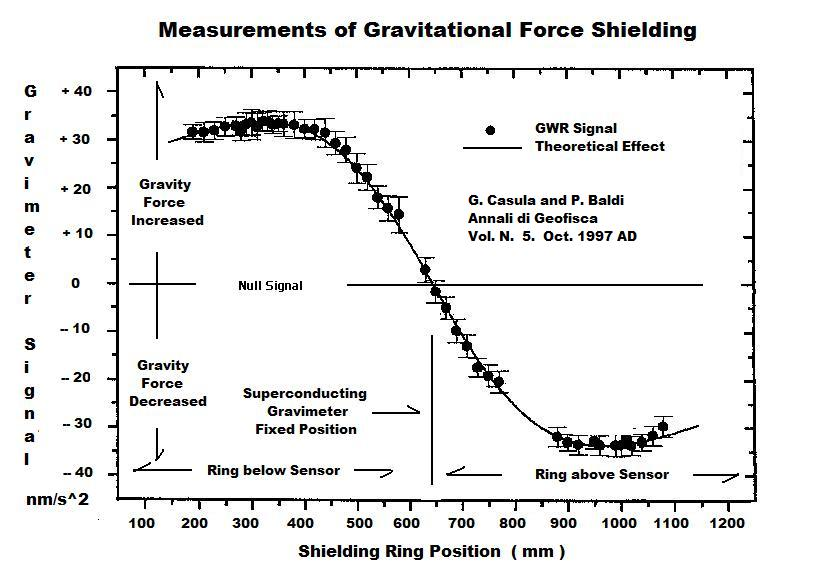Figure 3       Calibration Signal for Superconducting Gravimeter GWR, 1997 from
http://www.earth-prints.org/bitstream/2122/1569/1/12%20casula.pdf  .

This particular calibration chart, Fig. 3, was selected because it presents the complete data curve for the vertical  motion of the ring calibration mass.  It is clear that when the shielding ring mass is above the gravimeter sensor the force of gravity is reduced,...and when the ring mass is lowered below the sensor the apparent weight  force is increased.
This indicates that if identical rings were placed in the above and below shielding positions at the same time, the lower ring would mask a very large percentage of the shielding due to the above ring,...and therefore, very little or no shielding action would be found.
This is essentially the problem that Dr. Q. Majorana encountered when he shielded the above and below positions at the same time for his 1920 shielding tests. His delta g shielding test should have resulted in a reading of at least 30 microGals, instead of the recorded low value of ~ 1 microGal.

It is seen that when the ring is above the sensor the calibration test is successfully achieving the intended goals of Dr. Q. Majorana's shielding project of 1920. A replication of Majorana's experiment should be conducted without the shielding below the test mass. It will be necessary for any new Majorana replication experiments to follow the ring test procedure and make separate measurements for shielding above and below the test mass (gravimeter sensor).  Two gravimeter shielding calibration tests by others are: Varga 1994 and Casula 1997.

 < Prev Pg Double Force Page Contents Site Page List < Site Contents Bottom GvR 15

The original purpose of these ring calibration projects was to provide a very accurate calibration for these gravimeters. One does not necessarily have to know the true first cause of gravity in order to perform an accurate calibration of a gravimeter. However;...this ring calibration method and the resulting data are repeatable, valuable and clear evidence that the cause of gravity is a radiation and shadowing system.                                ***

### The Sun's and Jupiter's Gravity and Mass Anomaly

The radiation and shielding system and math presented here indicate that:

•  The material Sun contributes 4 percent or less of the mass and gravitational force displayed by the center of the Solar system.
•  Jupiter contributes only 43 percent or less of the mass and gravitational force for its location.

The individual magnetic fields surrounding the Sun and Jupiter shield the gravitational radiation in the same manner as solid matter and this explains the bodies "apparent" anomalous excess mass.
All planets and Suns larger than Earth provide total shielding of gravitational radiation. In this work these large planets are called black shadow planets, since their projected shadow contains no radiation from the direction through the planet. These large planets, except Jupiter, all have the same maximum potential radiation pressure of  86.6 million PSI at the surface, and all have the same surface gravity of 1.141 g.

In order for the Solar system center to provide enough gravitational force to maintain the planets in orbit, Nasa data indicates that the Sun's surface gravity must be 27.96 g.  Since the maximum surface gravity in our radiation and shielding system is 1.141 g, the cross-sectional area of the Sun would have to be 24.5 times larger than it's visual cross-sectional area in order to produce the required force.

The magnetic field of the Solar system center shields gravity in the same manner as solid matter and provides the large area of shielding required for the total force towards the solar system center. This gravitational shielding via a magnetic field produces a true gravitational force that is not related to magnetic "attraction".
If the gravitational shadow of the magnetic field is a black shadow shield, it's projected area must be 24.5 times larger than the visual Sun, with a total diameter five times that of the Sun. If it is a gray shadow shield the area must increase accordingly.

Since the magnetic shielding surrounds the spherical visual Sun, the Sun itself may exist within a field of very low gravity and therefore have a surface gravity approaching zero. With low gravity, inertia and pressure at the surface of the visible Sun, the large looping mass ejections seem predictable and may be due to meteor impacts and their molecular disintegration. Since the Sun appears to have no atmosphere or atmospheric pressure, meteors would arrive at the visual surface intact after traveling through the magnetic gravitational shield. Energetic disintegration of the meteor may occur as it enters the area of zero gravitational pressure and near zero mechanical pressure.

 < Prev Pg Double Force Page Contents Site Page List < Site Contents Bottom GvR 16

If the Sun has near zero gravity at its visual surface, a zero gravity core boundary may exist below, near the surface and encompass most of the Sun's material. However, if the visual Sun is a complete plasma it may not have enough gravitational shielding to produce a zero gravity core.
The material within all zero gravity cores is expected to be a nucleon and atom plasma within a field of incoherent prime radiation. Incoherent radiation produces no gravitational force. If the surface gravity of the Sun is at one percent of the maximum, the internal mechanical pressure must also be only one percent of the maximum gravitational radiation potential pressure of our Solar System as listed in the next paragraph.

Unlike the black shadow Sun and Jupiter, the other black shadow planets have maximum gravity at their surface and a large zero gravity core. The zero gravity core exists because all gravitational radiation is absorbed before reaching the planet center. This core would support the mechanical weight pressure of the outer layers and the atmosphere, but the core material would not exhibit weight or inertia.
The isotropic mechanical pressure within the large planets zero gravity cores would equal the maximum gravitational radiation potential pressure of the our Solar System, 86.6 million PSI,  [ 6.09 meg Kgf/cm^2 , 587.1 GPa ] . Theoretically, there will be no gradient of the mechanical pressure within the boundary of this zero gravity core.

Jupiter, a black shadow planet, is the only planet in our solar system that exists within a magnetic field which is large enough to shield gravitational radiation. NASA data predicts that Jupiter's surface gravity must be 2.54 g in order to maintain it's moons in their present orbits. Since the maximum surface gravity in our Solar System is 1.141 g, the cross-sectional area of Jupiter would have to be 2.23 times larger than it's visual cross-sectional area in order to produce the required force.
The existence of Jupiter's atmosphere demonstrates that unlike the Sun, Jupiter has some surface gravity. This indicates that the magnetic field only provides partial shielding. Therefore the body of Jupiter will provide a black shadow area in the center of the magnetic gray shadow area. The magnetic field then provides an extended gray shadow area sufficient to produce the total required force.
The web article on this site,  Magnetism as Radiant Whirlpools , presents the concept of magnetism as a soft unstable form of matter.

Current literature and NASA continue to refer to the large planets as gas planets. The persistent scars left on Jupiter by the Shoemaker Levy comets is evidence that it is not a ball of gas. A radiation and shadowing system predicts that the large planets have a surface just as solid as the small planets. The apparent low density exists because a large portion of the mass of each large planet is internally shielded from gravitational and inertial radiation.
A review and understanding of the mechanics of a radiation and shielding system of gravity will aid in studying the Sun and Jupiter shielding and the mass anomalies presented here.
The radiation and shielding system of gravity is presented in the paper, "Radiant Pressure Model of Remote Forces",  available at the URL    https://energy-gravity.comgrav11.htm  on this web site.

 < Prev Pg Double Force Page Contents Site Page List < Site Contents Bottom GvR 17

### Addendum A:        Developing the Gravitational Shielding Equations

The rate, d(D)/dy, of decrease of gravitational force (D) due to shielding by material depth (y) is:

Eq. 3          d(D) / dy = (-) Lm x D     or     (1 / D) x d(D) = ( - )Lm x dy

By separation of variables and integrating both sides we obtain the natural Log Equation 3a where C is a constant of integration.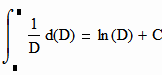Eq. 3a         Logn (D) = (- Lm x y )+ C1        Natural log base e

Solving for D we obtain Equation 3b:

Eq. 3b         D =  C2 x e^(-Lm x y)

On the surface (y) is equal to zero, therefore the constant C2 is equal to Do.  So Equation 4 results.

Eq. 4          D = Do x e^(-Lm x y)

Solving for Lm

Eq. 5          ln(D/Do) =  -(Lm x y)

Eq. 6         Lm =  - ln(D/Do) / y                      ***

 < Prev Pg Double Force Page Contents Site Page List < Site Contents Bottom GvR 18

### Addendum B:    Light Shielding by Depth of Lake Water.

( I )  Capital I is the Light intensity, a variable that decreases with depth below the surface.
( Io )     is the Light Intensity, a constant at the surface.
( Lm )   is the absorption ratio and is determined by the the clarity of the water.
( y )      is the depth in meters of water shielding the light.
( e )      is the base for natural logarithms.

The loss of intensity at any given depth in meters (y) is proportional to the reduced intensity (I) at that depth. This is shown in Equation 3.  Lm is the absorption ratio and its average value depends on the clarity of the water.

Equation 3                                   d(I)/dy = (-) Lm x I

This second equation shows that the light intensity , (I)  decays as a natural exponential function with the depth, (y), of shielding .

Equation 4                                    I = Io x e^( - Lm x y )

For the clear lakes of Northern Michigan the shielding rate (Lm) is 0.15068 when the depth is measured in meters. This value of shielding reduces the light to 50 percent at a depth of 4.6 meters, (15 ft.).  At a depth of 30.56 meters, (99 ft.), the light has been reduced to one percent .  The math steps for obtaining Eq. 4 from Eq. 3 is provided in Addendum A ,  above.     ***

 < Prev Pg Double Force Page Contents Site Page List < Site Contents Bottom GvR 19

In order to mathematically model the forces of the solar system, the following simplifying assumptions are made for the system's unknown physical properties.

• A homogeneous distribution of matter exists in each system body.
• The average maximum "effective" mass density occurs at the surface due to shielding of the interior.
• The effective mass density, inertial and weight of all system bodies decreases with depth due to shielding.
• All planets larger than Earth have a large inner core with zero gravity, mass and inertia, due to complete shielding by the upper layers.
• There are no known gas planets.
• For small planets a zero gravity point exists at the center due to a balance of radiation from opposing sides.
• The gravitational absorption rate increases with planet size due to backscatter of radiation.
• The physical constants found for our solar system may not apply to our Universe.
• The galactic magnetic field encompassing our system appears to shield and reduce our maximum gravitational pressure to a value below the maximum of the galaxy.
• There are no attractive forces and no action at a distance forces within this radiation shielding system of gravitation, electrostatics, magnetism and cohesion of particulate and nucleons.
• A planets physical properties of weight, mass, density, inertia and moment of inertia, are all subject to the effects of shielding.
• Gravity is a force per unit mass and the dimensional units of grams force per gram mass will be the basic units used for this study of gravity,...i.e.,... 1g  =  1gf / gm .
Using 1g as an acceleration will be avoided in the calculations for this radiant force model.        ***

 < Prev Pg Double Force Page Contents Site Page List < Site Contents Bottom GvR 20

### Reference:  Grav. Constants

P. Varga et. al. 1994  Abstract
Laboratory calibration of Lacoste–Romberg type gravimeters by using a heavy cylindrical ring
http://www3.interscience.wiley.com/journal/119253482/abstract

G. Casula and P. Baldi,  Annali di Geofisca, vol. XL, N. 5. Oct. 1997
Computation of Improved tidal parameters at the gravimeter station of Brasimone
http://www.earth-prints.org/bitstream/2122/1569/1/12%20casula.pdf

Q. Majorana’s Experiments on Gravitational Absorption
Prof. Giorgio Dragoni's.  Bologna University, Bologna, Italy
http://sci-ed.org/Conference-2004/Proceedings/Dragoni.pdf

http://sci-ed.org/Conference

"Force Doubling Paradox of Gravitational Attraction"

***

 < Prev Pg Double Force Page Contents Site Page List < Site Contents Bottom GvR 21

### Web Site Page List

Home
Index
TOC
Pressure    TOC
Gravity
Shield    TOC
Gravity
Anomaly     TOC
Resolve
Anomaly    TOC
vsEnergy     TOC
Inertia &
Magnetism    TOC
Field
Propulsion   TOC
Images     TOC
Create
Momentum    TOC
Force
Interactions   TOC
Light Speed vs
Relativity     TOC
False Constant
Lite Spd    TOC
Double Force
TOC
Gravity's
Constants     TOC
Create
Energy     TOC
Letters
Web Site
Images
Page List

R F
Energy     TOC
Energy via
Ionosphere     TOC
Demo of
RF Energy  TOC
RF Energy
Index   TOC

 < Prev Pg Double Force Page Contents Site Page List < Site Contents Top Next Pg > Create Energy

Derive Constants: Fini      GvR 22